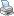Collapse Sidebar

 Students / SubjectsExperiments > MarketLink > Duopoly Market ExperimentsPrinter Friendly

Duopoly Market Experiments

In a duopoly, the price is below the monopoly price and above the competitive price; the duopoly quantity is above the monopoly quantity and below the competitive quantity. The prices and exchange quantity from experiments can be compared to the predicted price and quantity from the Cournot model.

To add the configuration for a double auction duopoly market to your profile, click the button below.

To add the configuration for a posted offer duopoly market to your profile, click the button below.

Duopoly model price and quantity predictions

Market Inverse Demand

The market inverse demand is p = D-1(Q) = 45 - Q, where the market output Q is the sum of the outputs q1 by Firm 1 and q2 by Firm 2.

Marginal cost for Firm 1

The marginal cost for Firm 1 is MC1(q1) = 3 + 2 q1

and the cost function for Firm 1 is C1(q1) = 3 q1 + q12.

Profit function for Firm 1

The profit function for Firm 1 can be determined from its revenue, which is p q1 = D-1(Q) q1, and its cost C1(q1). Combining these results in p1(q1, q2) = (45 - (q1 + q2)) q1 - 3 q1 - q12.

Response function for Firm 1

The derivative of the profit function is d p1/dq1 = 42 - 4 q1 - q2.

When this is equated to 0 and solved for q1 the result is so the first-order condition is q1* = 21/2 - ¼ q2.

Response function for Firm 2

By following a similar procedure for Firm 2 to the one above for Firm 1, the response function q2* = 21/2 - ¼ q1 for Firm 2 is obtained.

Equilibrium outputs and price

The equilibrium outputs are obtained by solving the two equations q1* = 21/2 B - ¼ q2 and q2* = 21/2 - ¼ q1 simultaneously. This results in

q1* = q2* = 42/5.

The equilibrium price is therefore p* = D-1(84/5) = 28.2.